# Introduction

To this point, we have found interpolating polynomials which pass through n points. Here we will see how we can not only match points, but also derivatives and higher derivatives at points.

This technique also generalizes for interpolating non-polynomial functions or points in more than two dimensions.

# Background

Useful background for this topic includes:

• The Vandermonde matrix.

# Problem

Given n constraints which match either values (0th derivative), 1st derivatives, 2nd derivatives, etc, up to the (n − 1)st derivative such that for any 1 ≤ kn, there are k constraints which are derivatives of order k − 1 or less.

# Assumptions

There are no conflicting or equivalent constraints.

# Process

For each of n constraints on the x-value xi which has the kth derivative, define the entry in the Vandermonde matrix V = (vi,j) as:

where y is the set of contrsaints. The solution to this system of linear equations are the coefficients of the interpolating polynomial.

For example, given five constraints, the rows for constraints at xi of 0th, 1st, 2nd, 3rd, and 4th derivatives would be

• xi4     xi3     xi2     xi     1
• 4xi3     3xi2     2xi     1     0
• 12xi2     6xi     2     0     0
• 24xi     6     0     0     0
• 24     0     0     0     0

respectively.

To be completed.

# Examples

1. Find the polynomial which has the value 1, slope 0.5, and concavity 0.25 at the point x = 2.

The system of linear equations is

c=10.50.25" >

which has a solution c = (0.125, 0, 0.5)T. A plot is shown in Figure E1.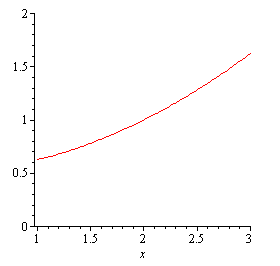Figure E1. The polynomial which has value, slope, and concavity of 1, 0.5, and 0.125 at x = 2.

2. Find the polynomial which has value 1 at x = 1, slope 2 at x = 2, and concavity 3 at x = 3.

The system of linear equations is

c=123" >

Notice that the point at which the concavity is fixed is irrelevant.

This has the solution c = (1.5, -4, 3.5)T. A plot is shown in Figure E2.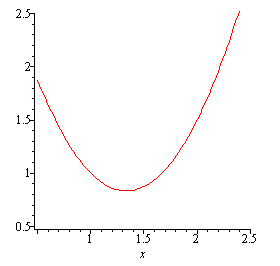Figure E2. The polynomial which has value, slope, and concavity of 1, 0.5, and 0.125 at x = 1, 2, and 3, respectively.

3. Find the polynomial which has value and slope 1 at x = 0 and value and slope e at x = 1. Plot the solution and plot the error |p3(x) − ex|.

The system of linear equations is

which has the solution c = (3 − e, 2e - 5, 1, 1)T. The plot of the polynomial p3(x) = (3 − e)x3 + (2e − 5)x2 + x + 1 and the error as compared to the exponential function are shown in Figure E3a and E3b, respectively.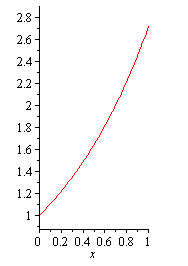Figure E3a. The polynomial with values and slopes 1 at x = 0 and e at x = 1.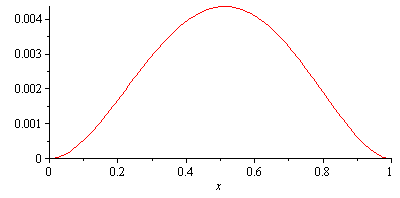Figure E3b. The difference of the approximation with the exponential function.

4. Find the polynomial which matches the value, slope, and concavity of the sine function at x = 1 and x = 2.

The system of linear equations is

which has the solution c = (0.0039113, 0.012864, -0.17864, -0.0011108, 1.0074, -0.0029858)T. The plot and error is shown in Figures E4a and E4b, respectively.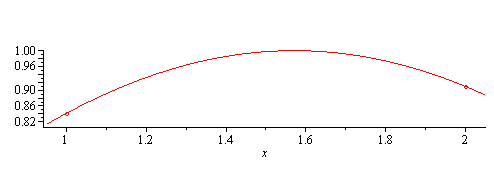Figure E4a. The polynomial with values, slopes, and concavity matching the sine function at x = 1 and x = 2.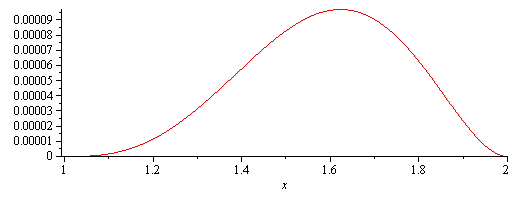Figure E4b. The difference of the approximation with the sine function.

# Applications to Engineering

This is used for splines but can also be used in interpolating points found as solutions to initial-value problems.